# Low-Noise Amplifier Stability Concept to Practical Considerations, Part 1

### 要約

Part one of a three-part series. Presents a brief overview of transmission lines and power gain definitions. Provides the basic background needed to design amplifiers for stability.

The design of small-signal, low-noise RF amplifiers is a step-by-step logical procedure with an exact solution for each problem. To aid the designer, many books offer complete schematics with component values "adaptable to the needs of a given application." But such circuits are designed for a specific set of operating conditions that may not correspond to your requirements. The absence of design procedures in these texts can leave readers helpless when attempting to adapt their circuit to a particular set of operating conditions.

This article takes the opposite approach. It presents a design process in which detailed, step-by-step procedures allow you to choose the LNA you want and operate it under any realistic operating conditions. You no longer have to adapt someone else's schematic to your specifications. Instead, you can create your own RF low-noise amplifiers and optimize them for a targeted application.

This article (Part 1) begins the discussion with a brief overview of transmission lines and a reminder on RF power gain definitions.

In Part 2, we jump into the RF aspect of low-noise amplifiers by examining stability (the tendency for oscillation), impedance matching, and general amplifier design, using the scattering parameters (s-parameters) as design tools.

Part 3 completes the series by presenting application examples. The first shows how to match an LNA in the maximum available gain condition. The second deals with an LNA matched in the constant desired gain condition. The third exercise stresses the importance of matching a potentially unstable LNA in its stable area.

### Transmission-Line Background (Reflection and Transmission)

Voltage, current, or power emanating from a source impedance Zs and delivered to a load ZL can be regarded as the sum of incident and reflected waves traveling in opposite directions along a transmission line of characteristic impedance Zo. If ZL equals Zo exactly, the incident wave is totally absorbed in the load and produces no reflected wave.

If ZL differs from Zo, some of the incident wave is not absorbed in the load, but is reflected back toward the source. If the source impedance Zs equals Zo, the reflected wave from the load is absorbed in the source and no further reflection occurs. For Zs not equal to Zo, a portion of the reflected wave from the load is re-reflected from the source back toward the load. For a lossless transmission line, this process repeats indefinitely.

The degree of mismatch between Zo and ZL (or Zs) determines the amount of incident wave reflected. The ratio of reflected wave to incident wave is known as the reflection coefficient, and is simply a measure of the quality of the match between the transmission line and the terminating impedance. The reflection coefficient Γ is a complex quantity expressed in polar form as a magnitude and an angle:As matching between the characteristic impedance of the transmission line and the terminating impedance improves, the reflected wave becomes smaller. As a result, the reflection coefficient (Γ) decreases. When the match is perfect there is no reflected wave, and Γ equals zero. If the load impedance is an open or short circuit, no incident power can be absorbed in the load, and all must be reflected back toward the source. In that case, Γ is 1. The normal range of magnitude for Γ is between zero and one.

For reflection coefficients greater than one, the magnitude of the wave reflected from the load impedance should be greater than that of the incident wave to that load. It follows, therefore, that the load in question must be a source of power. This concept is useful in designing an oscillator, but at the input network of an amplifier it represents bad news. Reflection coefficients can be expressed in terms of the impedances under consideration. For example, the reflection coefficient at the load can be expressed as: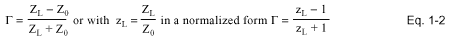For impedance matching in microwave and RF networks, the source and load impedances are often expressed in terms of the source reflection coefficient (Γs) and load reflection coefficient (ΓL). Incident and reflected waves are represented by wave flow graphs. Flow graphs let you build a graphic based on linear relations among different variables in the network, and they help in rapidly constructing a transfer function between two points in the network.

Each variable is represented by a node in the graph, and the different nodes are linked together by directive paths that give the relations between related and unrelated variables. Paths are directed from the node representing the independent variable to those representing the dependant variables, and to each path is assigned a gain related to the reflection coefficient linking the two variables.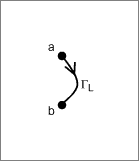Variables a and b are complex values associated with the incident and reflected waves. The presence of variable b (reflected wave) depends on the presence of variable a (incident wave).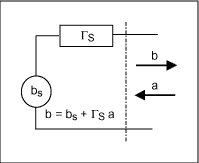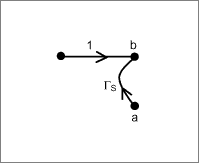Γs = b/a is the reflection coefficient related to the power source when bs = 0.
bs = b is the incident wave from the power source when it supplies a 100% matched load.

### S-Parameters and the Two-Port Network

By inserting a two-port network between source and load in the circuit of Figure 1-3a, we produce the circuit of Figure 2-1. The following may be said for any traveling wave that originates at the source:

• A portion of the wave that originates at the source and is incident on the two-port device (a1) will be reflected (b1), and another portion will be transmitted through the two-port device.
• A fraction of the transmitted signal is then reflected from the load and becomes incident on the output port of the two-port device (a2).
• A portion of the signal (a2) is then reflected from the output port back toward the load (b2), while a fraction is transmitted through the two-port device to the source.It is obvious from the above discussion that any traveling wave present in the circuit is composed of two components. For instance, the traveling wave components flowing from the output of the two-port device to the load consist of the portion of a2 reflected from the output of the two-port device, and the portion of a1 transmitted through the two-port device. Similarly, the traveling wave flowing from the input of the two-port device back toward the source consists of the portion of a1 reflected from the input and the fraction of a2 transmitted through the two-port device. If we set these observations in equation form we get the following:

b1 = S11a1 + S12a2
b2 = S21a1 + S22a2.

Where:
S11 = the input reflection coefficient
S12 = the reverse transmission coefficient
S21 = the forward transmission coefficient
S22 = the output reflection coefficient with ΔS = (S11S22 - S21 S12)

If we set a2 equal to zero, then S11 = b1/a1 | a2 = 0.
If we set a1 equal to zero, then S22 = b2/a2 | a1 = 0.

By definition, a reflected wave divided by an incident wave is equal to the reflection coefficient, as noted above for the input and output of a two-port network. A two-port network (LNA) can be characterized completely by its scattering parameters (S-parameters). With these parameters, you can calculate potential instabilities (tendency to oscillate), maximum available gain, input and output impedances, and transducer gain. You can also calculate the optimum source and load impedances, either for simultaneous conjugate matching, or simply to help choose specific source and load impedances for a specified transducer gain.

Figure 2-2a shows a two-port network connection for available gain definition. Γin is the two-port input reflection coefficient, and the output is terminated in ΓL. Γout is the two-port output reflection coefficient, as long as the input is terminated with Γs.The flow graph for a loaded two-port network (Figure 2-2b) shows a closed loop that allows starting from one node and returning to that node without going through it twice. The precise physical significance of these loops is illustrated by our two-port network (an LNA for example), whose output is closed on a load ΓL (Figure 2-3).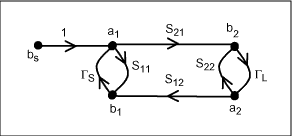To define the wave b1 reflected back to the input, we just follow the different paths leading from a1 to b1. Possible paths are the following:

S11, S21ΓLS12, S21ΓLS22ΓLS12, S21ΓLS22ΓLS22ΓLS12, ...

It appears that the loop S22Γ2 represents a multiple reflection due to the two-port network reflection coefficient between the output and the mismatched load.The network input reflection coefficient for the two ports is:When we close the two-port network input on a source impedance, the reflection coefficient seen from the output is (Figure 2-4):### Gain for Two-Port Networks

Waves a and b are directly related to the concept of power. Unlike the low-frequency domain in which current and voltage gain are of primary interest, in the RF and microwave domains only power gain is considered. The different gains used by two-port devices are defined below, but first consider some facts regarding electrical power.

### Maximum Available Power

The amount of power delivered to a load is easily determined, as shown by the connection of a source bs, Γs to a load termination ΓL (Figure 3-1). The power delivered by a source to a matched load is defined as the maximum available power (maximum power transferred) from the source when Γs = ΓL*. For these conditions, half the power is dissipated in the source and half is dissipated (transmitted) into the load. Figure 3-1 includes a flow graph.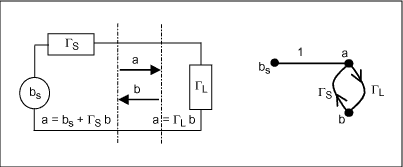The reflection seen from the source is: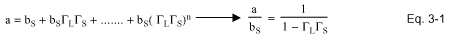Power transmitted to the load can be expressed asWhen ΓL = Γs*, the source can provide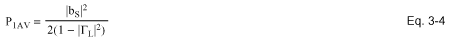The asterisk indicates the complex conjugate, in which magnitude is the same but the angle has the opposite sign.

### Two-Port Network Power Gain

Also defined as the operating gain or desired gain, it is the specific gain we want from a two-port network (LNA) for a particular application: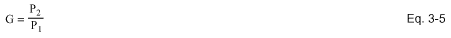Where P2 is power dissipated in the two-port output's load:Where P1 is the power absorbed by the two-port inputFrom the Figure 2-4 flow chart, we can easily extrapolate how b2 is related to a1: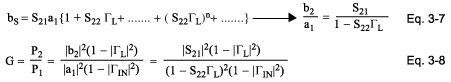WithTwo-port network power gain (operating or desired) is:### Maximum Available Gain for Two-Port Network

The maximum gain available from a two-port network is a particular case of the transducer gain obtained when ΓL* = ΓOUT. For a two-port network, the maximum available gain GAV is defined as the ratio of power available at the output to power available from the source, mathematically expressed as GAV = P2AV/P1AV. P2AV is the available output power, and P1AV is the power available from the source. Because maximum available gain can indicate whether an LNA has enough gain for the task, the calculation of this parameter is useful as a preliminary screening criteria for LNAs.

The initial wave bs2 seen from the two-port network output (Figure 2-4) is expressed as:At the two-port input, the source is able to supply (Figure 2-2b and Figure 2-3):Power available at the two-port output is: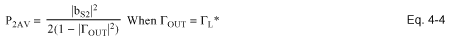Expressed as a function of the two-port network's input source,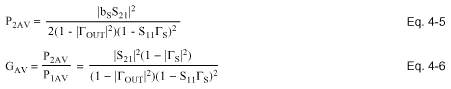As shown by Eq. (4-7):

ΓOUT = S22 + (S21ΓSS12 / 1 - S11ΓS

the maximum available gain factor depends on the source termination Γs and the two-port s-parameters. Maximum available gain depends on the two-port output being conjugately matched to ΓL. Thus, ΓL = Γout*. As shown in Eq. 2-2, Γout is a function of ΓS and the two-port parameters:The power available at the two-port output load is a function of power delivered to the two-port input, and power delivered to the two-port input depends on the mismatch between Γs and Γin. To determine power available from the source, you must terminate the source with a complex-conjugate load.

### Transducer Gain

Transducer gain (the gain term most often referenced in RF-amplifier design) is defined as the output power P2 delivered to a load by a source, divided by the maximum power available from the source. Transducer gain includes the effects of impedance matching at the input and output, as well as the contribution made by the LNA to the overall gain of the amplifier stage. Transducer gain neglects resistive losses in the components.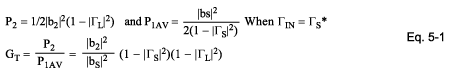To complete the transducer gain equation, define bs (the source initial wave) as a function of bs2, the initial wave seen from the two-port output (Figure 2-4), and b2 as the wave fed back from the load ΓL to the two-port network output.As shown in Eq. 2-2, ΓOUT is a function of Γs and the two-port parameters:Stability and maximum available gain (MAG) are two of the more important considerations in choosing a two-port network (LNA) for use in amplifier design. As used here, stability measures the tendency of an LNA to oscillate. Maximum available gain is a figure of merit for the LNA, which indicates the maximum theoretical power gain you can expect from the device when it is conjugately matched to its source and load impedances.

Transducer gain for a two-port network is: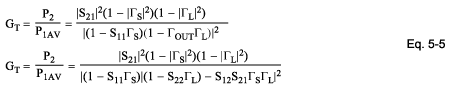### 参考資料

Bowick, Chris. RF Circuit Designs. Howard W. Sams & Co. Inc., a publishing subsidiary of ITT.

A similar version of this article appeared in the October 2001 issue of Microwaves and RF magazine.